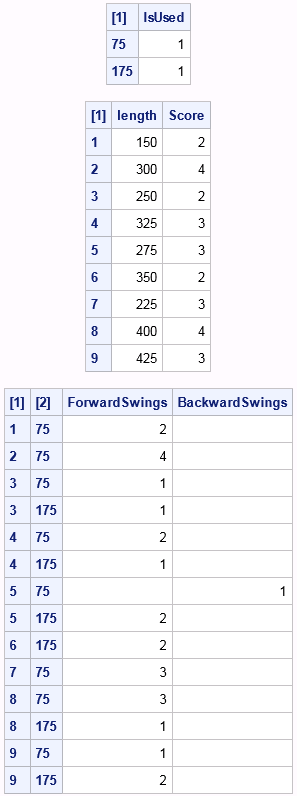Here's a golf puzzle from Sam Loyd:

Everybody is playing golf now, and even the lazy ones who a few weeks ago declared how much pleasanter it was to swing in a shady hammock, have caught the golf fever and are chasing the ball around the golf links. I am not much of a golfer, but I have met a genius who has a winning system based on mathematics. He says: “Just cultivate two strokes of different lengths, one a drive, the other an approach, and play directly toward the hole so that a combination of the two distances will get you there.”

What should be the proper lengths of strokes to learn that would make possible the lowest score on a nine-hole course, of 150 yards, 300 yards, 250 yards, 325 yards, 275 yards, 350 yards, 225 yards, 400 yards, and 425 yards? The ball must go the full length on each stroke, but you may go beyond the hole with either stroke, then play back toward the hole. All strokes are on a straight line toward the hole.

You can solve this problem by using mixed integer linear programming (MILP). The binary decision variable IsStroke[s]is 1 if stroke s is used, and 0 otherwise. The integer decision variable ForwardSwings[h,s] represents the number of times, on hole h, stroke s is used in the forward direction. Similarly, the integer decision variable BackwardSwings[h,s] represents the number of times, on hole h, stroke s is used in the backward direction. So for each hole h, the sum of s * ForwardSwings[h,s] minus the sum of s * BackwardSwings[h,s] must equal the length of h. Two sets of constraints link (no pun intended) the integer variables to the binary variables: if ForwardSwings[h,s] > 0 or BackwardSwings[h,s] > 0, the constraints force IsStroke[s]= 1. The score for a given hole is the total number of swings (forward or backward) used on that hole, and the objective is to minimize the total score across all holes. Hint: no more than five swings are needed on any hole.

The following PROC OPTMODEL statements formulate and solve the problem. Note the use of the GCD function to reduce the set of possible strokes to multiples of 25, the greatest common divisor of the hole lengths.

```data hole_data; input length @@; datalines; 150 300 250 325 275 350 225 400 425 ;   proc optmodel; /* declare sets and parameters and read data */ set HOLES; num length {HOLES}; read data hole_data into HOLES=[_N_] length;   /* without loss of optimality, take possible strokes to be multiples of the gcd */ num gcd = gcd(of length[*]); put gcd=; set STROKES = gcd..max {h in HOLES} length[h] by gcd;   /* IsUsed[s] = 1 if stroke s is used; 0 otherwise */ var IsUsed {STROKES} binary;   /* ForwardSwings[h,s] = number of times, on hole h, stroke s is used in forward direction */ var ForwardSwings {h in HOLES, s in STROKES} integer >= 0 <= 5;   /* BackwardSwings[h,s] = number of times, on hole h, stroke s is used in backward direction */ var BackwardSwings {h in HOLES, s in STROKES} integer >= 0 <= 5;   /* use exactly two strokes */ con Cardinality: sum {s in STROKES} IsUsed[s] = 2;   /* Score[h] = number of forward or backward swings used on hole h */ impvar Score {h in HOLES} = sum {s in STROKES} (ForwardSwings[h,s] + BackwardSwings[h,s]);   /* minimize total number of swings */ min TotalScore = sum {h in HOLES} Score[h];   /* make each hole */ con MakeHole {h in HOLES}: sum {s in STROKES} s * (ForwardSwings[h,s] - BackwardSwings[h,s]) = length[h];   /* if ForwardSwings[h,s] > 0 then IsUsed[s] = 1 */ con LinkForward {h in HOLES, s in STROKES}: ForwardSwings[h,s] <= ForwardSwings[h,s].ub * IsUsed[s];   /* if BackwardSwings[h,s] > 0 then IsUsed[s] = 1 */ con LinkBackward {h in HOLES, s in STROKES}: BackwardSwings[h,s] <= BackwardSwings[h,s].ub * IsUsed[s];   /* call MILP solver */ solve;   /* print various parts of solution */ print {s in STROKES: IsUsed[s].sol > 0.5} IsUsed; print length Score; print {h in HOLES, s in STROKES: ForwardSwings[h,s].sol > 0.5} ForwardSwings {h in HOLES, s in STROKES: BackwardSwings[h,s].sol > 0.5} BackwardSwings; quit;```

On my machine, the solver immediately finds that the minimum total score is 26, attainable by using strokes of length 75 and 175, since IsUsed = IsUsed = 1 in the optimal solution found.For example, the score on hole 5 using these strokes is Score = 3, with ForwardSwings[5,175] = 2 and BackwardSwings[5,75] = 1. And you can check that 2 * 175 - 1 * 75 = 275, the length of hole 5, as desired.

It turns out that a different set of two strokes, besides {75,175}, can also yield an optimal total score of 26. Can you find them?

Share